# Quantum Numbers

Quantum numbers can be used to describe the trajectory and the movement of an electron in an atom. The quantum numbers of all the electrons in a given atom, when combined, must comply with the Schrodinger equation.

## What are Quantum Numbers?

The set of numbers used to describe the position and energy of the electron in an atom are called quantum numbers. There are four quantum numbers, namely, principal, azimuthal, magnetic and spin quantum numbers.

The values of the conserved quantities of a quantum system are given by quantum numbers. Electronic quantum numbers (the quantum numbers describing electrons) can be defined as a group of numerical values which provide solutions that are acceptable by the Schrodinger wave equation for hydrogen atoms.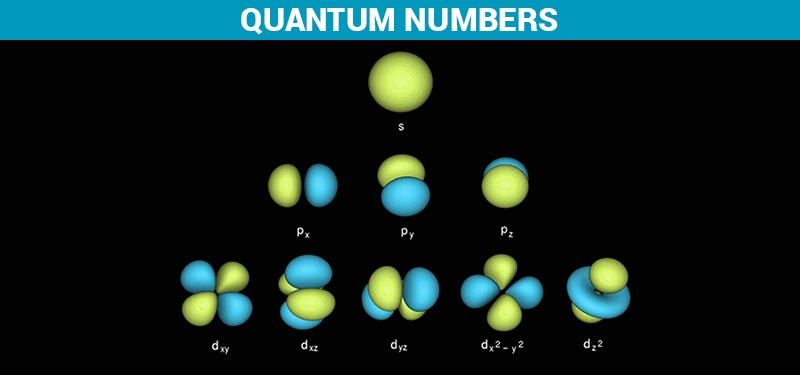Quantum Numbers and Atomic Orbitals

Four quantum numbers can be used to completely describe all the attributes of a given electron belonging to an atom, these are:

• Principal quantum number, denoted by n.
• Orbital angular momentum quantum number (or azimuthal quantum number), denoted by l.
• Magnetic quantum number, denoted by ml.
• The electron spin quantum number, denoted by ms.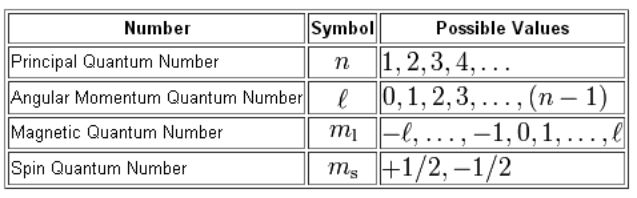The Four Quantum Numbers that Describe an Electron

## Recommended Videos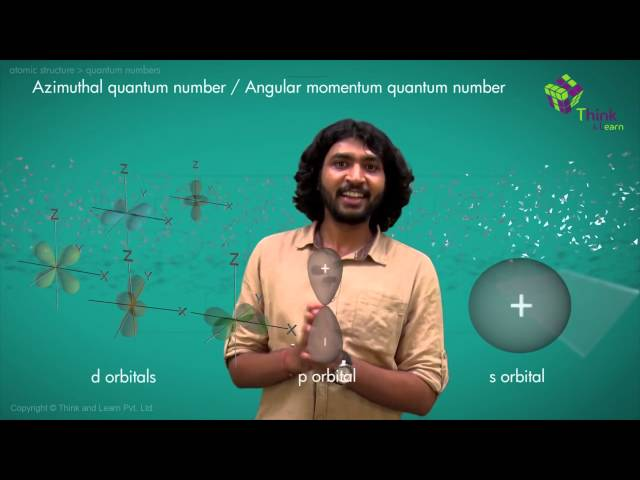When the characteristics of an electron must be described in compliance with the Schrodinger wave equation, a total of four quantum numbers are used. A brief description of each of these numbers in the set of four quantum numbers that describe the unique quantum state of an electron in atomic physics can be found below.

## Principal Quantum Number

• Principal quantum numbers are denoted by the symbol ‘n’. They designate the principal electron shell of the atom. Since the most probable distance between the nucleus and the electrons is described by it, a larger value of the principal quantum number implies a greater distance between the electron and the nucleus (which, in turn, implies a greater atomic size).
• The value of the principal quantum number can be any integer with a positive value that is equal to or greater than one. The value n=1 denotes the innermost electron shell of an atom, which corresponds to the lowest energy state (or the ground state) of an electron.
• Thus, it can be understood that the principal quantum number, n, cannot have a negative value or be equal to zero because it is not possible for an atom to have a negative value or no value for a principal shell.
• When a given electron is infused with energy (excited state), it can be observed that the electron jumps from one principle shell to a higher shell, causing an increase in the value of n. Similarly, when electrons lose energy, they jump back into lower shells and the value of n also decreases.
• The increase in the value of n for an electron is called absorption, emphasizing the photons or energy being absorbed by the electron. Similarly, the decrease in the value of n for an electron is called emission, where the electrons emit their energy.

## Azimuthal Quantum Number (Orbital Angular Momentum Quantum Number)

• The azimuthal (or orbital angular momentum) quantum number describes the shape of a given orbital. It is denoted by the symbol ‘l’ and its value is equal to the total number of angular nodes in the orbital.
• A value of the azimuthal quantum number can indicate either an s, p, d, or f subshell which vary in shapes. This value depends on (and is capped by) the value of the principal quantum number, i.e. the value of the azimuthal quantum number ranges between 0 and (n-1).
• For example, if n =3, the azimuthal quantum number can take on the following values – 0,1, and 2. When l=0, the resulting subshell is an ‘s’ subshell. Similarly, when l=1 and l=2, the resulting subshells are ‘p’ and ‘d’ subshells (respectively). Therefore, when n=3, the three possible subshells are 3s, 3p, and 3d.
• In another example where the value of n is 5, the possible values of l are 0, 1, 2, 3, and 4. If l = 3, then there are a total of three angular nodes in the atom.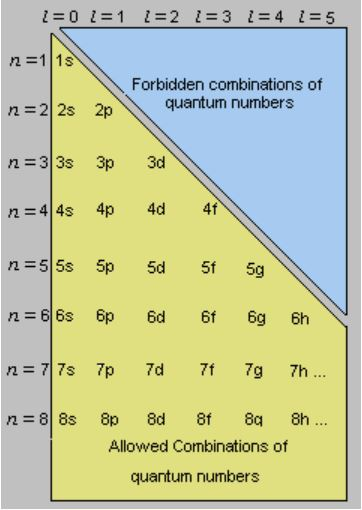Combinations of the Principal and Azimuthal Quantum Numbers

The allowed subshells under different combinations of ‘n’ and ‘l’ are listed above. It can be understood that the ‘2d’ orbital cannot exist since the value of ‘l’ is always less than that of ‘n’.

## Magnetic Quantum Number

The total number of orbitals in a subshell and the orientation of these orbitals are determined by the magnetic quantum number. It is denoted by the symbol ‘ml’. This number yields the projection of the angular momentum corresponding to the orbital along a given axis.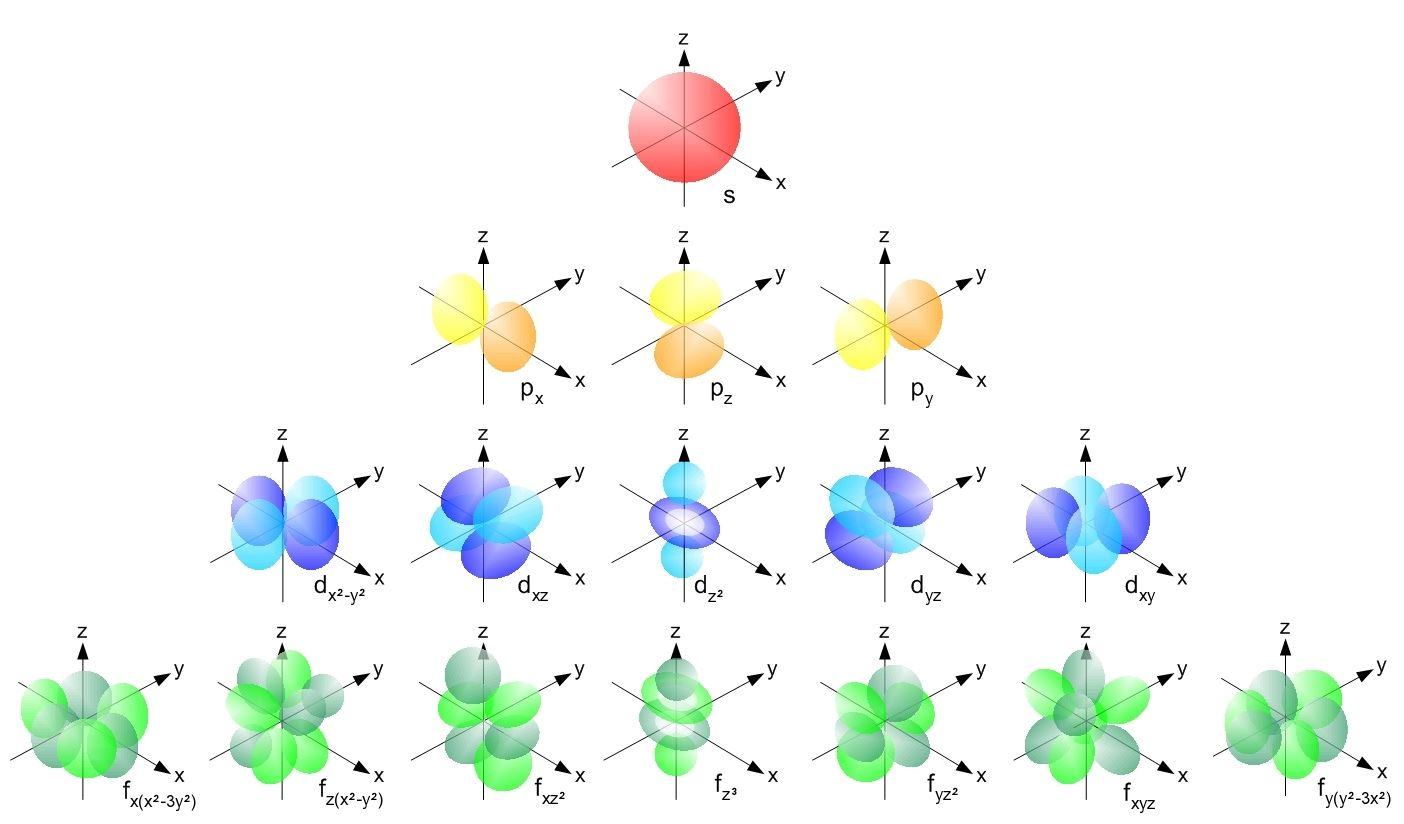Shapes of Orbitals (as per the corresponding Quantum Numbers)

The value of the magnetic quantum number is dependant on the value of the azimuthal (or orbital angular momentum) quantum number. For a given value of l, the value of ml ranges between the interval -l to +l. Therefore, it indirectly depends on the value of n.

For example, if n = 4 and l = 3 in an atom, the possible values of the magnetic quantum number are -3, -2, -1, 0, +1, +2, and +3.

 Azimuthal Quantum Number Value Corresponding Number of Orbitals (2l + 1) Possible Values of ml 0 (‘s’ subshell) 2*0 + 1 = 1 0 1 (‘p’ subshell) 2*1 + 1 = 3 -1, 0, and 1 2 (‘d’ subshell) 2*2 + 1 = 5 -2, -1, 0, 1, and 2 3 (‘f’ subshell) 2*3 + 1 = 7 -3, -2, -1, 0, 1, 2, and 3

The total number of orbitals in a given subshell is a function of the ‘l’ value of that orbital. It is given by the formula (2l + 1). For example, the ‘3d’ subshell (n=3, l=2) contains 5 orbitals (2*2 + 1). Each orbital can accommodate 2 electrons. Therefore, the 3d subshell can hold a total of 10 electrons.

## Electron Spin Quantum Number

• The electron spin quantum number is independent of the values of n, l, and ml. The value of this number gives insight into the direction in which the electron is spinning, and is denoted by the symbol ms.
• The value of ms offers insight into the direction in which the electron is spinning. The possible values of the electron spin quantum number are +½ and -½.
• The positive value of ms implies an upward spin on the electron which is also called ‘spin up’ and is denoted by the symbol ↑. If ms has a negative value, the electron in question is said to have a downward spin, or a ‘spin down’, which is given by the symbol ↓.
• The value of the electron spin quantum number determines whether the atom in question has the ability to produce a magnetic field. The value of ms can be generalized to ±½.

## Summary

In order to simplify the details of the four different quantum numbers that are related to atomic physics, a tabular column detailing their names, symbols, meanings, and possible values is provided below.

 Name and Symbol Meaning and Possible Values Principal quantum number, n Electron shell, n ≥ 1 Azimuthal quantum number, l Subshells (s=0, p=1, etc.) , (n-1) ≥ l ≥ 0 Magnetic quantum number, ml Total number and orientation of orbitals, l≥ml≥-l Electron spin quantum number, ms The direction of electron spin, ms = ±½

It is important to note that it is impossible for two electrons of the same atom to have exactly the same quantum state or exactly the same values of the set of quantum numbers, as per Hund’s rules.

## Solved Examples

### What are the Possible Subshells when n = 4? How Many Orbitals are Contained by Each of these Subshells?

When n = 4, the possible l values are 0, 1, 2, and 3. This implies that the 4 possible subshells are the 4s, 4p, 4d, and 4f subshells.

• The 4s subshell contains 1 orbital and can hold up to 2 electrons.
• The 4p subshell contains 3 orbitals and can hold up to 6 electrons.
• The 4d subshell contains 5 orbitals and can hold up to 10 electrons.
• The 4f subshell has 7 orbitals and can hold up to 14 electrons.

Thus, a total of 4 subshells are possible for n = 4.

### What are the Possible ml values for l = 4?

Since the value of the magnetic quantum number ranges from -l to l, the possible values of ml when l = 4 are: -4, -3, -2, -1, 0, 1, 2, 3, and 4.

## Frequently Asked Questions – FAQs

### Who proposed the principal quantum number?

The notion of energy levels and notation has been taken from the atom ‘s earlier Bohr model. Schrodinger ‘s equation evolved the concept from a two-dimensional flat Bohr atom to a three-dimensional model for wave motion. Where n = 1 , 2 , 3 is called the main quantity, and h is the constant of Planck.

### Why are there only 8 electrons in the outer shell?

The stability of an atom ‘s eight-electrons derives from the stability of the noble gases or the elder term of inert gases, also known as unreactive or noble gases. This law, however, is justified in the periodic table for second row elements whose outermost-shell capacity is 8 electrons.

### How do you find the principal quantum number?

The principal quantum number n value is the level of the central electronic shell (central level). All orbitals with the same n value are at the same key stage. All orbitals on the second main stage , for example, have a principal quantity of n=2.

### What are the principal energy levels?

In chemistry, an electron’s primary energy level refers to the shell or orbital in which the electron resides relative to the nucleus of the atom. The principal quantum number n denotes this level. Within a time of the periodic table the first element introduces a new key energy level.

### Which energy level has the least energy?

There is a single 1s orbital that can accommodate 2 electrons at the lowest energy level, the one nearest to the atomic core. There are four orbitals at the next energy level; a 2s, 2p1, 2p2 and a 2p3. Each of these orbitals can carry 2 electrons, so we can find a total of 8 electrons at this energy level.

### What is Quantum Energy?

Quantum, in mechanics, of energy, charge, angular momentum, or other physical property, discrete natural unit, or bundle. Photons, a concept often applied to quanta with other sources of electromagnetic radiation such as X rays and gamma rays, are certain particle-like packets of light.

### What is magnetic Polarisation?

The vector field that represents the density of permanent or induced magnetic dipole moments in a magnetic medium is magnetization or magnetic polarisation in classical electromagnetism. A pseudovector M is represented.

### What is the spin of an electron?

A quantum property of electrons is electron spin. It is an angular momentum shape. Instructors also equate electron spin to the planet rotating on its own axis every 24 hours as a teaching technique. If the electron spins on its axis clockwise, it is known as spin-up; spin-down is counterclockwise.

Test Your Knowledge On Quantum Numbers!

1. Rameshvalluri

I want to understand the hybridisation process in BeF2?

1. The BeF2 molecule features sp hybridization.

2. Jananee ammu

Plz give a simple definition of quantum number

1. In chemistry and quantum physics, quantum numbers describe values of conserved quantities in the dynamics of a quantum system.

2. Daniel Misiro.

I appreciated your lessons, I hope that you will keep in touch with me soon.

3. Mayuresh Nayak

What is “Au”

1. The element gold. Gold is element 79 and its symbol is Au. Though the name is Anglo Saxon, gold originated from the Latin Aurum, or shining dawn, and previously from the Greek.

4. Thank you so much Byjus for providing such a good study material with such a great explanation. It helps a lot.